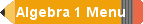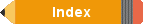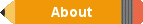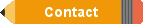Algebra 1 Common Core Standards
Algebra 1 Common Core Topics and Specifics

Number and Quantity
The Real Number System

CC.9-12.N.RN.1
Explain how the definition of the meaning of rational exponents follows from extending the properties of
integer exponents to those values, allowing for a notation for radicals in terms of rational exponents.

CC.9-12.N.RN.2
Rewrite expressions involving radicals and rational exponents using the properties of exponents.

CC.9-12.N.RN.3
Explain why the sum or product of two rational numbers is rational; that the sum of a rational number and an
irrational number is irrational; and that the product of a nonzero rational number and an irrational number is
irrational.

Quantities
CC.9-12.N.Q.1
Use units as a way to understand problems and to guide the solution of multi-step problems; choose and
interpret units consistently in formulas; choose and interpret the scale and the origin in graphs and data
displays.*

CC.9-12.N.Q.2
Define appropriate quantities for the purpose of descriptive modeling.*

CC.9-12.N.Q.3
Choose a level of accuracy appropriate to limitations on measurement when reporting quantities.*

* = Also a Modeling Standard

The Complex Number System

CC.9-12.N.CN.1
Know there is a complex number i such that i^2 = -1 and every complex number has the form a + bi with a and
b real.

CC.9-12.N.CN.2
Use the relation 2 = —1 and the commutative, associative, and distributive properties to add, subtract, and
multiply complex numbers.

CC.9-12.N.CN.3(+)
Find the conjugate of a complex number; use conjugates to find moduli and quotients of complex numbers.

CC.9-12.N.CN.7
Solve quadratic equations with real coefficients that have complex solutions.

CC.9-12.N.CN.9(+)
Know the Fundamental Theorem of Algebra; show that it is true for quadratic polynomials.

Algebra
Seeing Structure in Expressions

CC.9-12.A.SSE.1
Interpret expressions that represent a quantity in terms of its context.*

a. Interpret parts of an expression, such as terms, factors, and coefficients.

b. Interpret complicated expressions by viewing one or more of their parts as a single entity.

CC.9-12.A.SSE.2
Use the structure of an expression to identify ways to rewrite it.

CC.9-12.A.SSE.3
Choose and produce an equivalent form of an expression to reveal and explain properties of the quantity
represented by the expression.

a. Factor a quadratic expression to reveal the zeros of the function it defines.

b. Complete the square in a quadratic expression to reveal the maximum or minimum value of the function it
defines.

c. Use the properties of exponents to transform expressions for exponential functions.

CC.9-12.A.SSE.4
Derive the formula for the sum of a finite geometric series (when the common ratio is not 1), and use the
formula to solve problems.

Arithmetic with Polynomials and Rational Expressions

CC.9-12.A.APR.1
Understand that polynomials form a system analogous to the integers, namely, they are closed under the

CC.9-12.A.APR.2
Know and apply the Remainder Theorem: For a polynomial p(x) and a number a, the remainder on division by
x - a is p(a), so p(a) = 0 if and only if (x - a) is a factor of p(x).

CC.9-12.A.APR.3
Identify zeros of polynomials when suitable factorizations are available, and use the zeros to construct a
rough graph of the function defined by the polynomial.

CC.9-12.A.APR.4
Prove polynomial identities and use them to describe numerical relationships.

CC.9-12.A.APR.5(+)
Know and apply the Binomial Theorem for the expansion of (x + y)^n in powers of
x and y for a positive integer n, where x and y are any numbers, with coefficients determined for example by
Pascal’s Triangle. (The Binomial Theorem can be proved by mathematical induction or by a combinatorial
argument.)

CC.9-12.A.APR.6
Rewrite simple rational expressions in different forms; write a(x)/b(x) in the form q(x) + r(x)/b(x), where a(x), b
(x), q(x), and r(x) are polynomials with the degree of r(x) less than the degree of b(x), using inspection, long
division, or, for the more complicated examples, a computer algebra system.

Creating Equations

CC.9-12.A.CED.1
Create equations and inequalities in one variable and use them to solve problems. *

CC.9-12.A.CED.2
Create equations in two or more variables to represent relationships between quantities; graph equations on
coordinate axes with labels and scales.*

CC.9-12.A.CED.3
Represent constraints by equations or inequalities, and by systems of equations and/or inequalities, and
interpret solutions as viable or nonviable options in a modeling context. *

CC.9-12.A.CED.4
Rearrange formulas to highlight a quantity of interest, using the same reasoning as in solving equations.*

Reasoning with Equations and Inequalities

CC.9-12A.REI.1.
Explain each step in solving a simple equation as following from the equality of numbers asserted at the
previous step, starting from the assumption that the original equation has a solution. Construct a viable
argument to justify a solution method.

CC.9-12.A.REI.2
Solve simple rational and radical equations in one variable, and give examples showing how extraneous
solutions may arise.

CC.9-12.A.REI.3
Solve linear equations and inequalities in one variable, including equations with coefficients represented by
letters.

CC.9-12.A.REI.4
Solve quadratic equations in one variable.

a. Use the method of completing the square to transform any quadratic equation in x into an equation of the
form (x - p)^2 = q that has the same solutions. Derive the quadratic formula from this form.

b. Solve quadratic equations by inspection (e.g., for x^2 = 49), taking square roots, completing the square, the
quadratic formula and factoring, as appropriate to the initial form of the equation. Recognize when the
quadratic formula gives complex solutions and write them as a ± bi for real numbers a and b.

CC.9-12.A.REL5
Prove that, given a system of two equations in two variables, replacing one equation by the sum of that
equation and a multiple of the other produces a system with the same solutions.

CC.9-12.A.REL6
Solve systems of linear equations exactly and approximately (e.g., with graphs), focusing on pairs of linear
equations in two variables.

CC.9-12A.REI.7
Solve a simple system consisting of a linear equation and a quadratic equation in two variables algebraically
and graphically.

CC.9-12.A.REI.10
Understand that the graph of an equation in two variables is the set of all its solutions plotted in the
coordinate plane, often forming a curve (which could be a line).

CC.9-12.A.REI.11
Explain why the x-coordinates of the points where the graphs of the equations y = f(x) and y = g(x) intersect are
the solutions of the equation f(x) = g(x); find the solutions approximately, e.g., using technology to graph the
functions, make tables of values, or find successive approximations. Include cases where f(x) and for g(K)
are linear, polynomial, rational, absolute value, exponential, and logarithmic functions.*

CC.9-12.A.REI.12
Graph the solutions to a linear inequality in two variables as a half-plane (excluding the boundary in the case
of a Strict inequality), and graph the solution set to a system of linear inequalities in two variables as the
intersection of the corresponding half-planes.

Function

Interpreting Functions

CC.9-12F.IF.1
Understand that a function from one set (called the domain) to another set (called the range) assigns to each
element of the domain exactly one element of the range. If f is a function and x is an element of its domain,
then f(x) denotes the output of f corresponding to the input x. The graph of f is the graph of the equation y = f(x).

CC.9-1 2.F.IF.2
Use function notation, evaluate functions for ;nputs in their domains, and interpret statements that use
function notation in terms of a context.

CC.9-12.F.IF.3 Recognize that sequences are functions, sometimes defined recursively, whose domain is a
subset of the integers.

CC.9-12.F.IF.4 For a function that models a relationship between two quantities, interpret key features of
graphs and tables in terms of the quantities, and sketch graphs showing key features given a verbal
description of the relationship.*

CC.9-12.F.IF.5 Relate the domain of a function to its graph and, where applicable, to the quantitative
relationship it describes.*

CC.9-12.F.IF.6 Calculate and interpret the average rate of change of a function (presented symbolically or as
a table) over a specified interval. Estimate the rate of change from a graph.*

CC.9-12.F.IF.7
Graph functions expressed symbolically and show key features of the graph, by hand in simple cases and
using technology for more complicated cases.*

a. Graph linear and quadratic functions and show intercepts, maxima, and minima.

b. Graph square root, cube root, and piecewise- defined functions, including step functions and absolute
value functions.

c. Graph polynomial functions, identifying zeros when suitable factorizations are available, and showing end
behavior.

d. (+) Graph rational functions, identifying zeros and asymptotes when suitable factorizations are available,
and showing end behavior.

e. Graph exponential and logarithmic functions, showing intercepts and end behavior, and trigonometric
functions, showing period, midline, and amplitude.

CC.9-12.FJF.8
Write a function defined by an expression in different but equivalent forms to reveal and explain different
properties of the function.

a. Use the process of factoring and completing the square in a quadratic function to show zeros, extreme
values, and symmetry of the graph, and interpret these in terms of a context.

b. Use the properties of exponents to interpret expressions for exponential functions.

CC.9-12.F.IF.9
Compare properties of two functions each represented in a different way (algebraically, graphically,
numerically in tables, or by verbal descriptions).

Building Functions

CC.9-1 2.F.BF.1
Write a function that describes a relationship between two quantities.*

a. Determine an explicit expression, a recursive process, or steps for calculation from a context.

b. Combine standard function types using arithmetic operations.

c. (+) Compose functions.

CC.9-12.F.BF.2
Write arithmetic and geometric sequences both recursively and with an explicit formula, use them to model
situations, and translate between the two forms.*

CC.9-12.F.BF.3
Identify the effect on the graph of replacing f(x) by f(x) + kf(x), f(kx), and f(x + k) for specific values of k (both
positive and negative); find the value of k given the graphs. Experiment with cases and illustrate an
explanation of the effects on the graph using technology.

CC.9-12.F.BF.4
Find inverse functions.

a. Solve an equation of the form f(x) = c for a simple function f that has an inverse and write an expression for
the inverse.

b. (+) Verify by composition that one function is the inverse of another.

c. (+) Read values of an inverse function from a graph or a table, given that the function has an inverse.

d. (+) Produce an invertible function from a non-invertible function by restricting the domain.

CC.9-12.F.BF.5(+)
Understand the inverse relationship between exponents and logarithms and use this relationship to solve
problems involving logarithms and exponents.

CC.9-12.F.LE.1
Distinguish between situations that can be modeled with linear functions and with exponential functions. *

a. Prove that linear functions grow by equal differences over equal intervals, and that exponential functions
grow by equal factors over equal intervals.

b. Recognize situations in which one quantity changes at a constant rate per unit interval relative to another.

c. Recognize situations in which a quantity grows or decays by a constant percent rate per unit interval relative
to another.

CC.9-12.F.LE.2
Construct linear and exponential functions, including arithmetic and geometric sequences, given a graph, a
description of a relationship, or two input-output pairs (include reading these from a table).*

CC.9-12.F.LE.3
Observe using graphs and tables that a quantity increasing exponentially eventually exceeds a quantity
increasing linearly, quadratically, or (more generally) as a polynomial function.*

CC.9-1.F.LE.4
For exponential models, express as a logarithm the solution to ab = d where c, and dare numbers and the
base b is 2, 10, or e; evaluate the logarithm using technology. *

CC.9-12.F.LE.5
Interpret the parameters in a linear or exponential function in terms of a context. *

Trigonometric Functions

CC.9-12.F.TF.1
Understand radian measure of an angle as the length of the arc on the unit circle subtended by the angle.

CC.9-12.F.TF.2
Explain how the unit circle in the coordinate plane enables the extension of trigonometric functions to all real
numbers, interpreted as radian measures of angles traversed counterclockwise around the unit circle.

CC.9-12.F.TF.3(+)
Use special triangles to determine geometrically the values of sine, cosine, tangent for π/3, π/4 and π/6, and
use the unit circle to express the values of sine, cosines, and tangent for x, π + x, and 2 π - x in terms of their
values for x, where x is any real number.

CC.9-12.F.TF.4(+)
Use the unit circle to explain symmetry (odd and even) and periodicity of trigonometric functions.

CC.9-12.F.TF.5
Choose trigonometric functions to model periodic phenomena with specified amplitude, frequency, and
midline. *

CC.9-12.F.TF.6(+)
Understand that restricting a trigonometric function to a domain on which it is always increasing or always
decreasing allows its inverse to be constructed.

CC.9-1 2.F.TF.7(+)
Use inverse functions to solve trigonometric equations that arise in modeling contexts; evaluate the solutions
using technology, and interpret them in terms of the context.*

CC.9-12.F.TF.8
Prove the Pythagorean identity sin^2(Θ) + cos^2(Θ) = 1 and use it to calculate trigonometric ratios.

CC.9-12.F.TF.9(+)
Prove the addition and subtraction formulas for sine, cosine, and tangent and use them to solve problems.

Geometry
Congruence

CC.9-12.G.CO.1
Know precise definitions of angle, circle, perpendicular line, parallel line, and line segment, based on the
undefined notions of point, line, distance along a line, and distance around a circular arc.

CC.9-12.G.CO.2
Represent transformations in the plane using, e.g., transparencies and geometry software; describe
transformations as functions that take points in the plane as inputs and give other points as outputs.
Compare transformations that preserve distance and angle to those that do not (e.g., translation versus
horizontal stretch).

CC.9-12.G.CO.3
Given a rectangle, parallelogram. trapezoid, or regular polygon, describe the rotations and reflections that
carry it onto itself.

CC.9-12.G.CO.4
Develop definitions of rotations, reflections, and translations in terms of angles, circles, perpendicular lines,
parallel lines, and line segments.

CC.9-12.G.CO.5
Given a geometric figure and a rotation, reflection, or translation, draw the transformed figure using, e.g.,
graph paper, tracing paper, or geometry software. Specify a sequence of transformations that will carry a
given figure onto another.

CC.9-12.G.CO.6
Use geometric descriptions of rigid motions to transform figures and to predict the effect of a given rigid
motion on a given figure; given two figures, use the definition of congruence in terms of rigid motions to
decide if they are congruent

CC.9-12.G.CO.7
Use the definition of congruence in terms of rigid motions to show that two triangles are congruent if and only
if corresponding pairs of sides and corresponding pairs of angles are congruent.

CC.9-12.G.CO.8
Explain how the criteria for triangle congruence (ASA, SAS, and SSS) follow from the definition of congruence
in terms of rigid motions.

CC.9-12.G.CO.9
Prove geometric theorems about lines and angles.

CC.9-12.G.CO.1O

CC.9-12.G,CO.11

CC.9-12.G.CO.12
Make formal geometric constructions with a variety of tools and methods (compass and straightedge, string,
reflective devices, paper folding, dynamic geometry software, etc.).

CC.9-1 2.G.CO.1 3
Construct an equilateral triangle, a square, and a regular hexagon inscribed in a circle.

Similarity, Right Triangles, and Trigonometry

CC.9-12.G.SRT.1
Verify experimentally the properties of dilations given by a center and a scale factor:

a. A dilation takes a line not passing through the center of the dilation to a parallel line, and leaves a line
passing through the center unchanged.

b. The dilation of a line segment is longer or shorter in the ratio given by the scale factor.

CC.9-12.G.SRT.2
Given two figures, use the definition of similarity in terms of similarity transformations to decide if they are
similar; explain using similarity transformations the meaning of similarity for triangles as the equality of all
corresponding angles and the proportionality of all corresponding pairs of sides.

CC.9-12.G.SRT.3
Use the properties of similarity transformations to establish the AA criterion for two triangles to be similar.

CC.9-12.G.SRT.4

CC.9-12.G.SRT.5
Use congruence and similarity criteria for triangles to solve problems and prove relationships in geometric
figures.

CC.9-12.G.SRT.6
Understand that by similarity, side ratios in right triangles are properties of the angles in the triangle, leading
to definitions of trigonometric ratios for acute angles.

CC.9-12.G.SRT.7
Explain and use the relationship between the sine and cosine of complementary angles.

CC.9-12.G.SRT.8
Use trigonometric ratios and the Pythagorean Theorem to solve right triangles in applied problems.

CC.9-12.G.SRT.9(+)
Derive the formula A = 1/2 ab sin(C) for the area of a triangle by drawing an auxiliary line from a vertex
perpendicular to the opposite side.

CC.9-12.G.SRT.1O(+)
Prove the Laws of Sines and Cosines and use them to solve problems.

CC.9-12.G.SRT.11(+)
Understand and apply the Law of Sines and the Law of Cosines to find unknown measurements in right and
non-right triangles (e.g., surveying problems, resultant forces).

Circles

CC.9-12.G.C.1
Prove that all circles are similar.

CC.9-12.G.C.2
Identify and describe relationships among inscribed angles, radii, and chords.

CC.9-12.G.C.3
Construct the inscribed and circumscribed circles of a triangle, and prove properties of angles for a

CC.9-12.G.C.4(+)
Construct a tangent line from point outside a given circle to the circle.

CC.9-12.G.C.5 Derive using similarity the fact that the length of the arc intercepted by an angle is proportional
to the radius, and define the radian measure of the angle as the constant of proportionality; derive the formula
for the area of a sector.

Expressing Geometric Properties with Equation

CC.9-12.G.GPE.1
Derive the equation of a circle of given center and radius using the Pythagorean Theorem; complete the
square to find the center and radius of a circle given by an equation.

CC.9-12.G.GPE.2
Derive the equation of a parabola given a focus and directrix.

CC.9-12.G.GPE.3(+)
Derive the equations of ellipses and hyperbolas given the foci, using the fact that the sum or difference of
distances from the foci is constant.

CC.9-12.G.GPE.4
Use coordinates to prove simple geometric theorems algebraically.

CC.9-12.G.GPE.5
Prove the slope criteria for parallel and perpendicular lines and use them to solve geometric problems (e.g.,
find the equation of line parallel or perpendicular to a given line that passes through a given point).

CC.9-12.G.GPE.6
Find the point on a directed line segment between two given points that partitions the segment in a given ratio.

CC.9-12.G.GPE.7
Use coordinates to compute perimeters of polygons and areas of triangles and rectangles, e.g., using the
distance formula.*

Geometric Measurement and Dimension

CC.9-12.G.GMD.1
Give an informal argument for the formulas for the circumference of a circle, area of a circle, volume of a
cylinder, pyramid, and cone.

CC.9-12.G.GMD.2(+)
Give an informal argument using Cavalieri’s principle for the formulas for the volume of a sphere and other
solid figures.

CC.9-12.G.GMD.3
Use volume formulas for cylinders, pyramids, cones, and spheres to solve problems. *

CC.9-12.G.GMD.4
Identify the shapes of two- dimensional cross-sections of three-dimensional objects, and identify three-
dimensional objects generated by rotations of two-dimensional objects.

Modeling with Geometry

CC.9-12.G.MG.1
Use geometric shapes, their measures, and their properties to describe objects (e.g., modeling a tree trunk
or a human torso as a cylinder). *

CC.9-12.G.MG.2
Apply concepts of density based on area and volume in modeling situations (e.g., persons per square mile,
BTUs per cubic foot).*

CC.9-12.G.MG.3
Apply geometric methods to solve design problems (e.g., designing an object or structure to satisfy physical
constraints or minimize cost; working with typographic grid systems based on ratios).*

Statistics and Probability

Interpreting Categorical and Quantitative Data

CC.9-12.S.ID.1
Represent data with plots on the real number line (dot plots, histograms, and box plots). *

CC.9-12.S.ID.2
Use statistics appropriate to the shape of the data distribution to compare center (median, mean) arid spread
(interquartile range, standard deviation) of two or more different data sets.*

CC.9-12.S.ID.3 Interpret differences in shape, center, and spread in the context of the data sets, accounting
for possible effects of extreme data points (outliers).*

CC.9-12.S.ID.4
Use the mean and standard deviation of a data set to fit it to a normal distribution and to estimate population
percentages. Recognize that there are data sets for which such a procedure is not appropriate. Use
calculators, spreadsheets, and tables to estimate areas under the normal curve.*

CC.9-1 2.S.ID.5
Summarize categorical data for two categories in two-way frequency tables. Interpret relative frequencies in
the context of the data (including joint, marginal, and conditional relative frequencies). Recognize possible
associations and trends in the data.*

CC.9-12.S.ID.6
Represent data on two quantitative variables on a scatter plot, and describe how the variables are related.*

a. Fit a function to the data; use functions fitted to data to solve problems in the context of the data.

b. Informally assess the fit of a function by plotting and analyzing residuals.

c. Fit a linear function for a scatter plot that suggests a linear association.

CC.9-12.S.ID.7
Interpret the slope (rate of change) and the intercept (constant term) of a linear model in the context of the
data.*

CC.9-12.S.ID.8
Compute (using technology) and interpret the correlation coefficient of a linear fit.*

CC.9-12.S.ID.9
Distinguish between correlation and causation.*

Making Inferences and justifying Conclusions

CC.9.12.S.IC.1
Understand statistics as a process for making inferences about population parameters based on a random
sample from that population.*

CC.9-12.S.IC.2
Decide if a specified model is consistent with results from a given data- generating process, e.g., using
simulation.*

CC.9-12.S.IC.3
Recognize the purposes of and differences among sample surveys, experiments, and observational studies;
explain how randomization relates to each.*

CC.9-12.S.IC.4
Use data from a sample survey to estimate a population mean or proportion; develop a margin of error
through the use of simulation models for random sampling.*

CC.9-12.S.IC.5
Use data from a randomized experiment to compare two treatments; use simulations to decide if differences
between parameters are significant.*

CC.9-12.S.IC.6
Evaluate reports based on data.*

Conditional Probability and the Rules of Probability

CC.9-12.S.CP.1
Describe events as subsets of a sample space (the set of outcomes) using characteristics (or categories) of
the outcomes, or as unions, intersections, or complements of other events (“or,” “and,” “not”). *

CC.9-12.S.CP.2
Understand that two events A and B are independent if the probability of A and B occurring together is the
product of their probabilities, and use this characterization to determine if they are independent.*

CC.9-12.S.CP.3
Understand the conditional probability of A given B as F(A and B)/P(B), and interpret independence of A and B
as saying that the conditional probability of A given B is the same as the probability of A, and the conditional
probability of B given A is the same as the probability of B.*

CC.9-12.S.CP.4
Construct and interpret two-way frequency tables of data when two categories are associated with each
object being classified. Use the two-way table as a sample space to decide if events are independent and to
approximate conditional probabilities.*

CC.9-12.S.CP.5
Recognize and explain the concepts of conditional probability and independence in everyday language and
everyday situations.*

CC.9-12.S.CP.6
Find the conditional probability of A given B as the fraction of B’s outcomes that also belong to A, and interpret
the answer in terms of the model.*

CC.9-12.S.CP.7
Apply the Addition Rule, P(A and B) = P(A) + P(B) – P(A and B),and interpret the answer in terms of the model.*

CC.9-12.S.CP.8(+)
Apply the general Multiplication Rule in a uniform probability model, P(A and B) = P(A)P(B|A) = P(B)P(A|B), and
interpret the answer in terms of the model.*

CC.9-12.S.CP.9(+)
Use permutations and combinations to compute probabilities of compound events and solve problems.*

Using Probability to Make Decisions

CC.9-12.S.MD.3(+)
Develop a probability distribution for a random variable defined for a sample space in which theoretical
probabilities can be calculated; find the expected value.*

CC.9-12.S.MD.5(+)
Develop a probability distribution for a random variable defined for a sample space in which probabilities are
assigned empirically; find the expected value.*

CC.9-12.S.MD.6(+)
Use probabilities to make fair decisions (e.g., drawing by lots, using a random number generator).*

CC.9-12.S.MD.7(+)
Analyze decisions and strategies using probability concepts (e.g., product testing, medical testing, pulling a
hockey goalie at the end of a game).*